• +91 9971497814
• info@interviewmaterial.com

# Chapter 10 Straight lines Ex-10.1 Interview Questions Answers

### Related Subjects

Question 1 : Draw a quadrilateral in the Cartesian plane, whose vertices are (–4, 5), (0, 7), (5, –5) and (–4, –2). Also, find its area.

Let ABCD be the given quadrilateral with vertices A (–4, 5), B (0, 7), C (5, –5), and D (–4, –2).
Then, by plotting A, B, C, and D on the Cartesian plane and joining AB, BC, CD, and DA, the given quadrilateral can be drawn as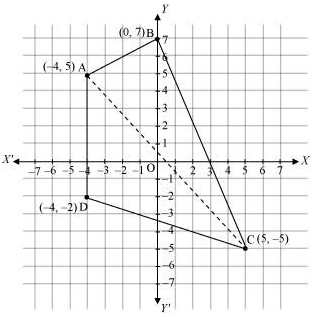To find the area ofquadrilateral ABCD, we draw one diagonal, say AC.

Accordingly, area (ABCD)= area (ΔABC) + area (ΔACD)

We know that the area ofa triangle whose vertices are (x1y1),(x2y2), and (x3y3)is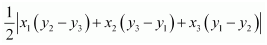Therefore, area of ΔABC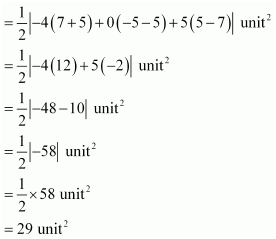Area of ΔACD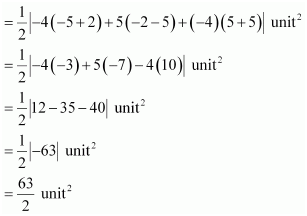Thus, area (ABCD)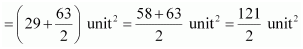Question 2 : The base of an equilateral triangle with side 2a lies along they y-axis such that the mid point of the base is at the origin. Find vertices of the triangle.

Let ABC be the given equilateral triangle with side 2a.
Accordingly, AB = BC = CA = 2a
Assume that base BC lies along the y-axis such that the mid-point of BC is at the origin.
i.e., BO = OC = a, where O is the origin.
Now, it is clear that the coordinates of point C are (0, a), while the coordinates of point B are (0, –a).
It is known that the line joining a vertex of an equilateral triangle with the mid-point of its opposite side is perpendicular.
Hence, vertex A lies on the y-axis.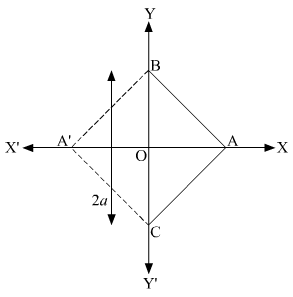On applying Pythagoras theorem to ΔAOC, we obtain

(AC)2 =(OA)2 + (OC)2

(2a)2 =(OA)2 + a2

4a2 – a2 =(OA)2

(OA)2 =3a2

OA =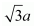Coordinates of point A =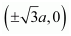Thus, the vertices ofthe given equilateral triangle are (0, a), (0, –a),and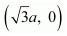or (0, a), (0, –a),and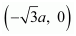.

Question 3 : Find the distance between P (x1, y1) and Q (x2, y2) when: (i) PQ is parallel to the y-axis, (ii) PQ is parallel to the x-axis.

Given:

Points P (x1,y1) and Q(x2, y2)

(i) WhenPQ is parallel to y axis then x1 = x2

So, the distance between P and Q is given by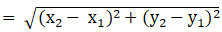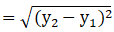= |y2 –y1|

(ii) WhenPQ is parallel to the x-axis then y1 = y2

So, the distance between P and Q is given by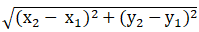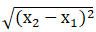= |x2 –x1|

Question 4 : Find a point on the x-axis, which is equidistant from the points (7, 6) and (3, 4).

Let us consider (a, 0) be the point on the x-axis that isequidistant from the point (7, 6) and (3, 4).

So,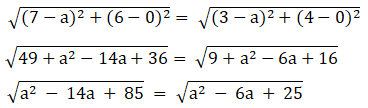Now, let us square on both the sides we get,

a2 – 14a + 85 = a2 – 6a + 25

-8a = -60

a = 60/8

= 15/2

Therequired point is (15/2, 0)

Question 5 : Find the slope of a line, which passes through the origin, and the mid-point of the line segment joining the points P (0, – 4) and B (8, 0).

The co-ordinates of mid-point of the line segment joining thepoints P (0, – 4) and B (8, 0) are (0+8)/2, (-4+0)/2 = (4, -2)

The slope ‘m’ of the line non-vertical line passing through thepoint (x1, y1) and

(x2, y2) is given by m = (y2 – y1)/(x2 – x1) where, x ≠ x1

The slope of the line passing through (0, 0) and (4, -2) is(-2-0)/(4-0) = -1/2

Therequired slope is -1/2.

Question 6 : Without using the Pythagoras theorem, show that the points (4, 4), (3, 5) and (–1, –1) are the vertices of a right-angled triangle.

The vertices of the given triangle are (4, 4), (3, 5) and (–1,–1).

The slope (m) of the line non-vertical line passing through thepoint (x1, y1) and

(x2, y2) is given by m = (y2 – y1)/(x2 – x1) where, x ≠ x1

So, the slope of the line AB (m1) = (5-4)/(3-4) = 1/-1 = -1

the slope of the line BC (m2) = (-1-5)/(-1-3) = -6/-4 = 3/2

the slope of the line CA (m3) = (4+1)/(4+1) = 5/5 = 1

It is observed that, m1.m3 =-1.1 = -1

Hence, the lines AB and CA are perpendicular to each other

giventriangle is right-angled at A (4, 4)

And the vertices of the right-angled ∆ are (4, 4), (3, 5) and(-1, -1)

Question 7 : Find the slope of the line, which makes an angle of 30° with the positive direction of y-axis measured anticlockwise.

We know that, if a line makes an angle of 30° with the positivedirection of y-axis measured anti-clock-wise , then the angle made by the linewith the positive direction of x- axis measure anti-clock-wise is 90° + 30° =120°

Theslope of the given line is tan 120° = tan (180° – 60°)

= – tan 60°

= –3

Question 8 : Find the value of x for which the points (x, – 1), (2, 1) and (4, 5) are collinear.

If the points (x, – 1), (2, 1) and (4, 5) are collinear, thenSlope of AB = Slope of BC

Then, (1+1)/(2-x) = (5-1)/(4-2)

2/(2-x) = 4/2

2/(2-x) = 2

2 = 2(2-x)

2 = 4 – 2x

2x = 4 – 2

2x = 2

x = 2/2

= 1

Therequired value of x is 1.

Question 9 : Without using distance formula, show that points (– 2, – 1), (4, 0), (3, 3) and (–3, 2) are the vertices of a parallelogram.

Let the given point be A (-2, -1) , B (4, 0) , C ( 3, 3) and D (-3, 2)

So now, The slope of AB = (0+1)/(4+2) = 1/6

The slope of CD = (3-2)/(3+3) = 1/6

Hence, slope of AB = Slope of CD

AB  CD

Now,

The slope of BC = (3-0)/(3-4) = 3/-1 = -3

The slope of AD = (2+1)/(-3+2) = 3/-1 = -3

Hence, slope of BC = Slope of AD

Thus the pair of opposite sides are quadrilateralare parallel, so we can say that ABCD is a parallelogram.

Hence the given vertices, A (-2, -1), B (4, 0), C(3, 3) andD(-3, 2) are vertices of a parallelogram.

Question 10 : Find the angle between the x-axis and the line joining the points (3, –1) and (4, –2).

The Slope of the line joining the points (3, -1) and (4, -2) isgiven by

m = (y2 –y1)/(x2 – x1) where, x ≠ x1

m = (-2 –(-1))/(4-3)

= (-2+1)/(4-3)

= -1/1

= -1

The angle of inclination of line joining the points (3, -1) and(4, -2) is given by

tan θ = -1

θ = (90° + 45°) = 135°

Theangle between the x-axis and the line joining the points (3, –1) and (4, –2) is135°.

Todays Deals### Chapter 10 Straight lines Ex-10.1 Contributorskrishan

Name:
Email:

# Latest News# 9000 interview questions in different categories